Newton's Laws - Lesson 3 - Newton's Second Law of Motion

# Free Fall and Air Resistance

In a previous unit, it was stated that all objects (regardless of their mass) free fall with the same acceleration - 9.8 m/s/s. This particular acceleration value is so important in physics that it has its own peculiar name - the acceleration of gravity - and its own peculiar symbol - g. But why do all objects free fall at the same rate of acceleration regardless of their mass? Is it because they all weigh the same? ... because they all have the same gravity? ... because the air resistance is the same for each? Why? These questions will be explored in this section of Lesson 3.

In addition to an exploration of free fall, the motion of objects that encounter air resistance will also be analyzed. In particular, two questions will be explored:

• Why do objects that encounter air resistance ultimately reach a terminal velocity?
• In situations in which there is air resistance, why do more massive objects fall faster than less massive objects?

To answer the above questions, Newton's second law of motion (Fnet = m•a) will be applied to analyze the motion of objects that are falling under the sole influence of gravity (free fall) and under the dual influence of gravity and air resistance.

###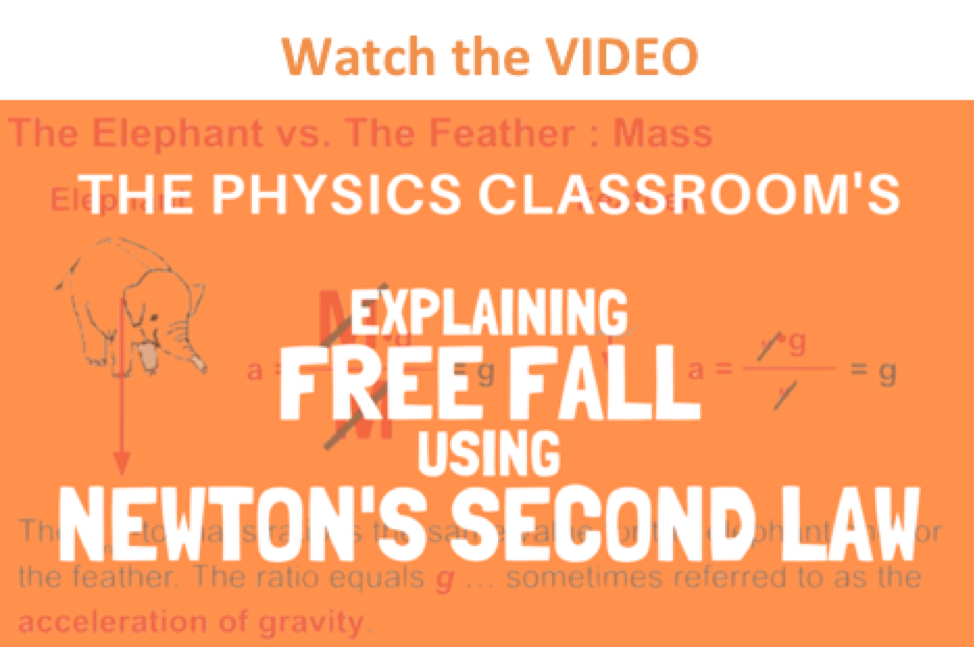Free Fall Motion

As learned in an earlier unit, free fall is a special type of motion in which the only force acting upon an object is gravity. Objects that are said to be undergoing free fall, are not encountering a significant force of air resistance; they are falling under the sole influence of gravity. Under such conditions, all objects will fall with the same rate of acceleration, regardless of their mass. But why? Consider the free-falling motion of a 1000-kg baby elephant and a 1-kg overgrown mouse.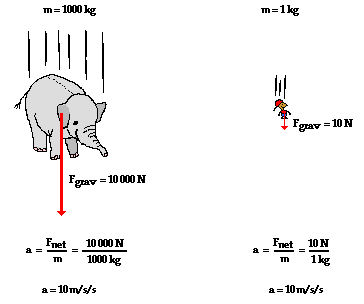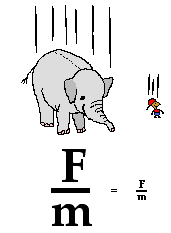If Newton's second law were applied to their falling motion, and if a free-body diagram were constructed, then it would be seen that the 1000-kg baby elephant would experiences a greater force of gravity. This greater force of gravity would have a direct effect upon the elephant's acceleration; thus, based on force alone, it might be thought that the 1000-kg baby elephant would accelerate faster. But acceleration depends upon two factors: force and mass. The 1000-kg baby elephant obviously has more mass (or inertia). This increased mass has an inverse effect upon the elephant's acceleration. And thus, the direct effect of greater force on the 1000-kg elephant is offset by the inverse effect of the greater mass of the 1000-kg elephant; and so each object accelerates at the same rate - approximately 10 m/s/s. The ratio of force to mass (Fnet/m) is the same for the elephant and the mouse under situations involving free fall.

This ratio (Fnet/m) is sometimes called the gravitational field strength and is expressed as 9.8 N/kg (for a location upon Earth's surface). The gravitational field strength is a property of the location within Earth's gravitational field and not a property of the baby elephant nor the mouse. All objects placed upon Earth's surface will experience this amount of force (9.8 N) upon every 1 kilogram of mass within the object. Being a property of the location within Earth's gravitational field and not a property of the free falling object itself, all objects on Earth's surface will experience this amount of force per mass. As such, all objects free fall at the same rate regardless of their mass. Because the 9.8 N/kg gravitational field at Earth's surface causes a 9.8 m/s/s acceleration of any object placed there, we often call this ratio the acceleration of gravity. (Gravitational forces will be discussed in greater detail in a later unit of The Physics Classroom tutorial.)### Look It Up!

The value of the gravitational field strength (g) is different in different gravitational environments. Use the Value of g widget below to look up the the gravitational field strength on other planets. Select a location from the pull-down menu; then click the Submit button.

### Investigate!

Even on the surface of the Earth, there are local variations in the value of g. These variations are due to latitude (the Earth isn't a perfect sphere; it buldges in the middle), altitude and the local geological structure of the region. Use the Gravitational Fields widget below to investigate how location affects the value of g.

As an object falls through air, it usually encounters some degree of air resistance. Air resistance is the result of collisions of the object's leading surface with air molecules. The actual amount of air resistance encountered by the object is dependent upon a variety of factors. To keep the topic simple, it can be said that the two most common factors that have a direct effect upon the amount of air resistance are the speed of the object and the cross-sectional area of the object. Increased speeds result in an increased amount of air resistance. Increased cross-sectional areas result in an increased amount of air resistance.Why does an object that encounters air resistance eventually reach a terminal velocity? To answer this questions, Newton's second law will be applied to the motion of a falling skydiver.In the diagrams below, free-body diagrams showing the forces acting upon an 85-kg skydiver (equipment included) are shown. For each case, use the diagrams to determine the net force and acceleration of the skydiver at each instant in time. Then use the button to view the answers.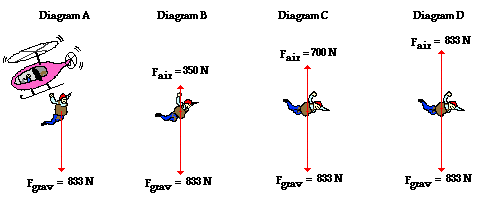The diagrams above illustrate a key principle. As an object falls, it picks up speed. The increase in speed leads to an increase in the amount of air resistance. Eventually, the force of air resistance becomes large enough to balances the force of gravity. At this instant in time, the net force is 0 Newton; the object will stop accelerating. The object is said to have reached a terminal velocity. The change in velocity terminates as a result of the balance of forces. The velocity at which this happens is called the terminal velocity.In situations in which there is air resistance, more massive objects fall faster than less massive objects. But why? To answer the why question, it is necessary to consider the free-body diagrams for objects of different mass. Consider the falling motion of two skydivers: one with a mass of 100 kg (skydiver plus parachute) and the other with a mass of 150 kg (skydiver plus parachute). The free-body diagrams are shown below for the instant in time in which they have reached terminal velocity.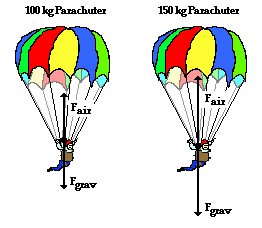As learned above, the amount of air resistance depends upon the speed of the object. A falling object will continue to accelerate to higher speeds until they encounter an amount of air resistance that is equal to their weight. Since the 150-kg skydiver weighs more (experiences a greater force of gravity), it will accelerate to higher speeds before reaching a terminal velocity. Thus, more massive objects fall faster than less massive objects because they are acted upon by a larger force of gravity; for this reason, they accelerate to higher speeds until the air resistance force equals the gravity force.

### Investigate!

The amount of air resistance an object experiences depends on its speed, its cross-sectional area, its shape and the density of the air. Air densities vary with altitude, temperature and humidity. Nonetheless, 1.29 kg/m3 is a very reasonable value. The shape of an object affects the drag coefficient (Cd). Values for various shapes can be found here. Use the What a Drag! widget below to explore the dependence of the air resistance force upon these four variables.

### We Would Like to Suggest ...Sometimes it isn't enough to just read about it. You have to interact with it! And that's exactly what you do when you use one of The Physics Classroom's Interactives. We would like to suggest that you combine the reading of this page with the use of our Skydiving Interactive and/or our Falling Bodies - 1D Interactive. You can find them in the Physics Interactives section of our website. The Skydiving Interactive allows a learner to explore the effect of mass, parachute size, and the initial height upon the experience of a skydiver.

Next Section: